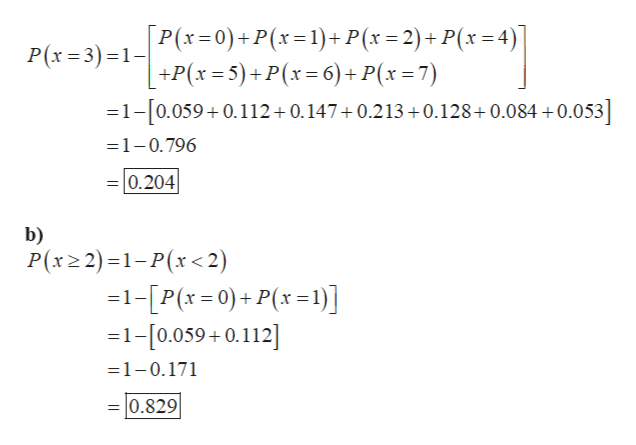# 14. The number of school related extracurricular activites per student are given below:X.           p(x)0          0.0591          0.1122         0.1473         ?4        0.2135        0.1286       0.0847        0.053Suppose that a week is randomly selected. Using the above table, finda.) p(x=3)  b.) p(x>2) c.) p(x<7) d.) p(1<x<5)

Question
31 views

14. The number of school related extracurricular activites per student are given below:

X.           p(x)

0          0.059

1          0.112

2         0.147

3         ?

4        0.213

5        0.128

6       0.084

7        0.053

Suppose that a week is randomly selected. Using the above table, find

a.) p(x=3)  b.) p(x>2) c.) p(x<7) d.) p(1<x<5)

check_circle

Step 1

a)

As the sum of the probabilities in the probability distribution table is 1,...help_outlineImage TranscriptioncloseР(х 3D3) -1-/P(r3D0)+ P(х%3D1) + Р(х%3D2)+P(х%3D4)| +P(х-5)+ P(х-6)+ P(х-7) =1-[0.059+ 0.112+0.147+0.213+0.128+0.084 +0.053] =1-0.796 =|0.204 b) Р(x>2) -1-Р(r <2) -1-[РP(*-0)+ P(*-1)] 1-[0.059+0.112] -1-0.171 =0.829 fullscreen

### Want to see the full answer?

See Solution

#### Want to see this answer and more?

Solutions are written by subject experts who are available 24/7. Questions are typically answered within 1 hour.*

See Solution
*Response times may vary by subject and question.
Tagged in

### Other